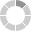# Celltone Demo

Celltone is a simple programming language for generative music composition, based on cellular automata. This is an interactive demo site where you can try Celltone without having to install it. Go here to find documentation, or get started with the examples.

## Write some code

15 sec## Debug

### Celltone output

`        `

### Timidity output

`        `

### Lame output

`        `

## Examples

• Bubblesort
```a = [12, 11, 9, 7, 5, 4, 2, 0]
x = [0, _, _, _, _, _, _, _]
x.velocity = 0
x.channel = 1

{a > a, x != 0} =>
{a = a, a = a}

<tempo> = 80
```
• Longer example
```a = [0, _, _, _]
b = [_, _, _]
c = [0, _, _, _, _, _, _, _]

a.transpose = 0
b.transpose = 5
c.transpose = 7

a.octava = 5
b.octava = 5
c.octava = 5

a.channel = 1
b.channel = 2
c.channel = 3

{<0> != _, <0> != _, <0> == 4} =>
{<0> = _, <2> = 7}
{<0> != _, <0> != _, <0> == 7} =>
{<0> = _, <2> = 9}
{<0> != _, <0> != _, <0> == 9} =>
{<0> = _, <2> = 0}
{<0> != _, <0> != _} =>
{<0> = _, <2> = <0>, <0> = 4, <1> = 7}

<tempo> = 100
<iterlength> = 16
```